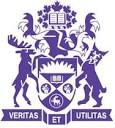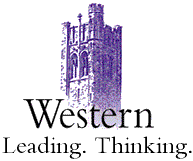### CS2214A: Discrete Structures for Computing, Fall 2020#### Lecture slides, recommended readings and supplementary materials

• Lectures:
• Lecture 1: [pdf] Introduction, motivation and work plan
• Lecture 2: [pdf] Propositional logic: syntax, semantics and truth table
• Tutorial:
• Tutorial 1 with solutions: [pdf] Predicate logic
• Suggested reading: [pdf] Professor Antonina Kolokolova's notes on propositional logic
• Lectures:
• Lecture 3: [pdf] Predicate Logic
• Tutorial:
• Tutorial 2 with solutions:: [pdf] Predicate logic
• Suggested reading: [pdf] Professor Antonina Kolokolova's notes on proof techniques
• Lectures:
• Lecture 4: [pdf] Proof techniques
• Tutorial:
• Tutorial 3 with solutions: [pdf] Logic and Proofs
• Suggested reading: [pdf] Professor Antonina Kolokolova's notes on proof techniques
• Lectures:
• Tutorial:
• Tutorial 4 with solutions:: [pdf]
• Suggested reading: [pdf] Chapter 4 of Mathematics for Computer Science by Eric Lehman, F Thomson Leighton and Albert R Meyer.
• Lectures:
• Lecture 5: [pdf] Functions, sequences, matrices
• Tutorial:
• Tutorial 5 with solutions: [pdf]
• Suggested reading: [pdf] Chapter 4 of Mathematics for Computer Science by Eric Lehman, F Thomson Leighton and Albert R Meyer.
• Lectures:
• Lecture 6: [pdf] Number theory
• Tutorial:
• Tutorial 6 with solutions: [pdf]
• Lectures:
• Lecture 7: [pdf] Number theory and cryptography
• Tutorial:
• Tutorial 7 with solutions: [pdf]
• Suggested reading: [pdf] Professor Chester Rebeiro's notes on RSA and Public Key Cryptography. For RSA, focus on Slides 10 to 15.
• Lectures:
• Lecture 8: [pdf] Induction and recursion
• Tutorial:
• Tutorial 8 with solutions: [pdf]
• Suggested reading: [pdf] Professor Antonina Kolokolova's notes on proof techniques

#### Tentative Assignment and Test Schedule

Posted/GivenDueWeight
Assignment 1 with solutions Mon Sept. 23Tu. Oct. 1, 23:555%
Assignment 2 with solutions Mon. Oct. 7Tu. Oct. 15, 23:555%
Assignment 3 with solutions Mon. Oct. 21Fr. Nov. 1, 23:555%
Midterm Exam with solutions Mon. Oct. 28 25%
Assignment 4 with solutions Mon. Nov. 11Tu. Nov. 19, 23:555%
Assignment 5 with solutions We. Nov. 20Mo. Dec. 1, 23:555%
Final ExamTBA 50%

#### Teaching Crew and Hours

• Instructor
Marc Moreno Maza, Professor
email: moreno@csd.uwo.ca

Lecture hours: Mondays (9:30-11:30, UCC-56), Tuesdays (11:30-12:30, SEB-1059) and Wednesdays (10:30 to 11:30, NS-7)

Office: Middlesex College, Room 327
Office hours: Tuesdays, 13:30 to 15:20

• Teaching Assistants
NameOffice/Tutorial HoursRoom
MOHAJERANI, Davood Thursday 13:30-14:30 MC 4A